# Cubes

#### • Probability

Cubes typically come in 1 cm (1 cm³) and 2 cm (8 cm³) sizes. They can be made of wood or plastic, link on all sides, some sides, or be smooth.

#### 1cm Cubes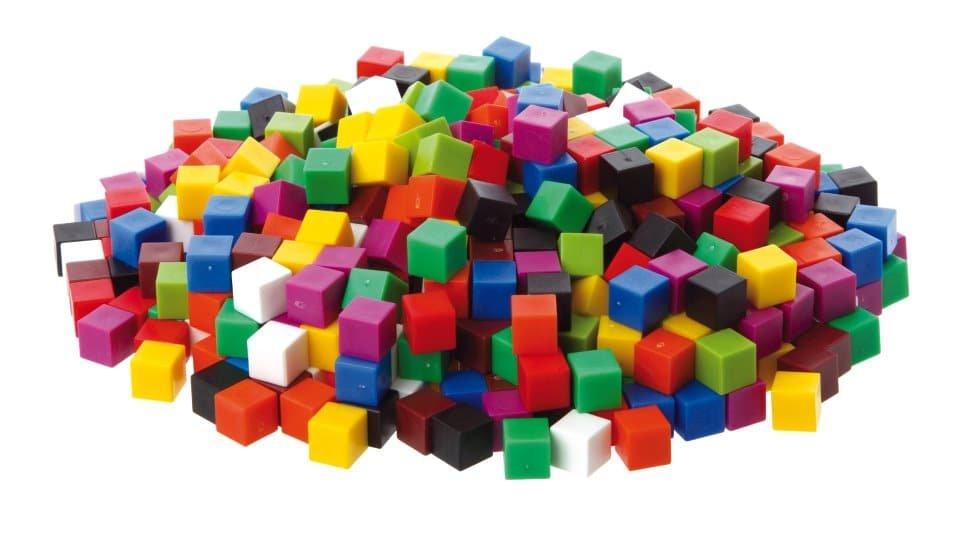#### Wooden Cubes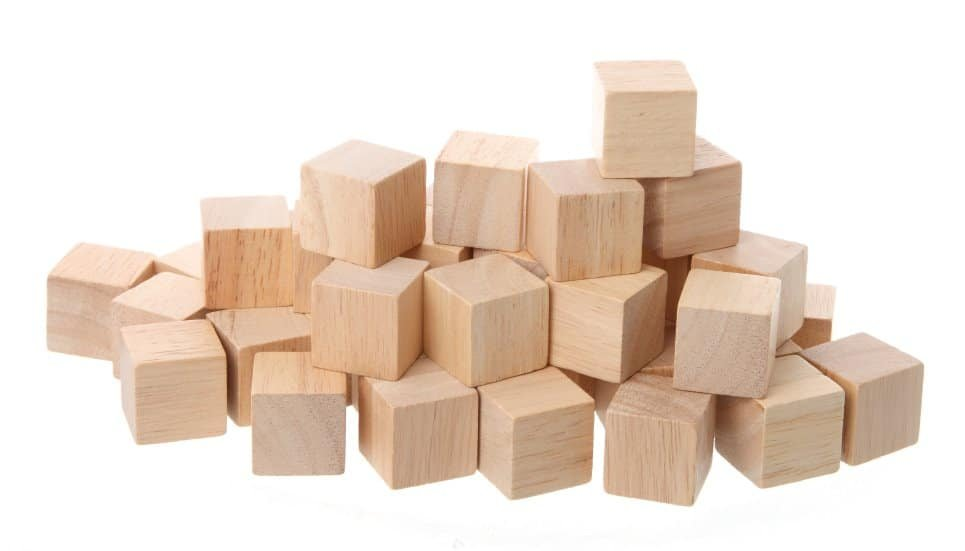#### 1cm Interlocking Cubes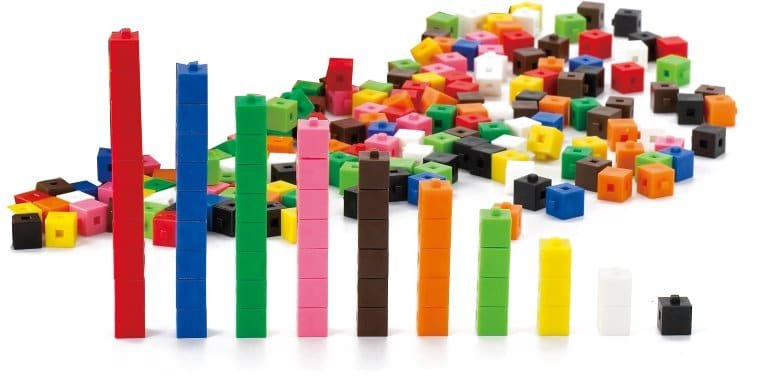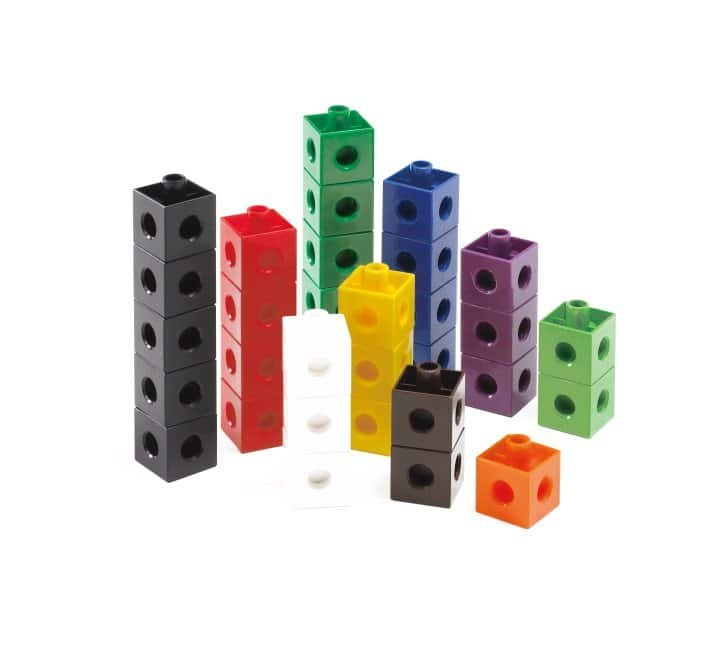### Cubes Videos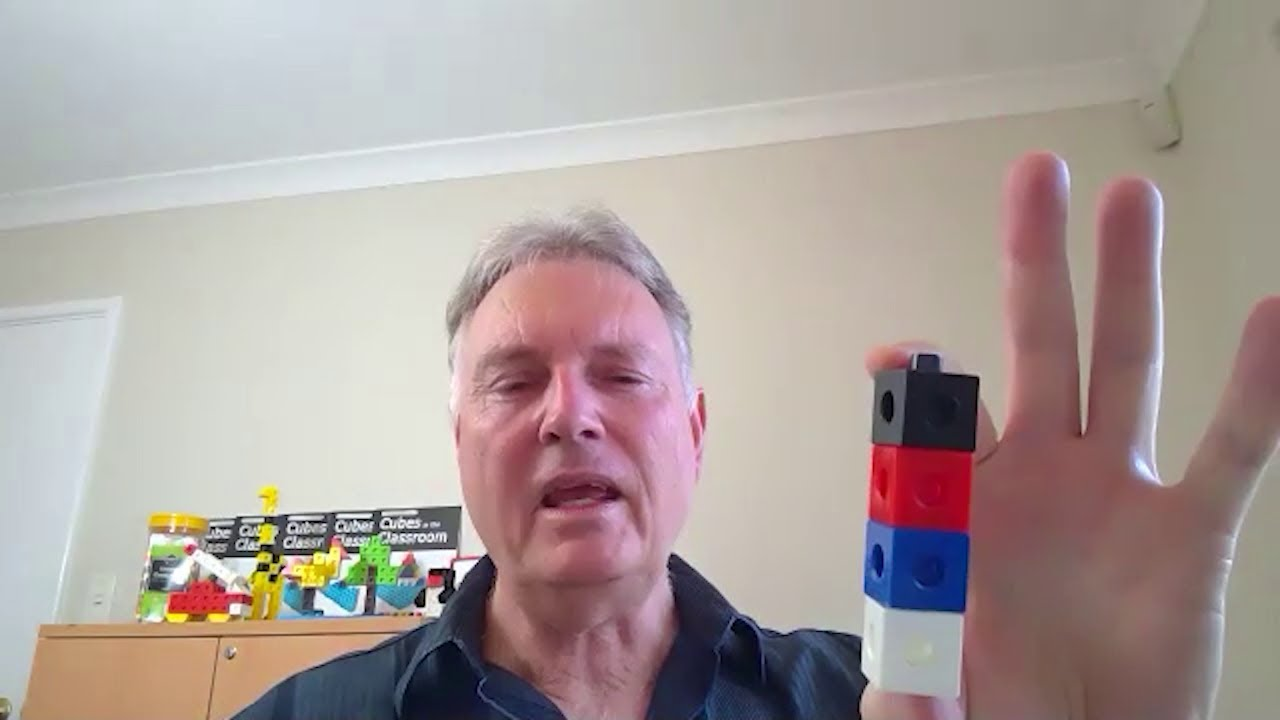0:00 – Webinar Intro
1:30 – Different types of Cubes
3:10 – Construction
7:30 – Partitioning, Commutative Property of Addition (turn around facts)
9:36 – Simple Game for Addition
11:50 – Patterning, Algebra foundations
17:20 – Cube Battleships
18:10 – Problem Solving
19:45 – Spatial thinking
23:10 – 3D Object Construction / 2D Plans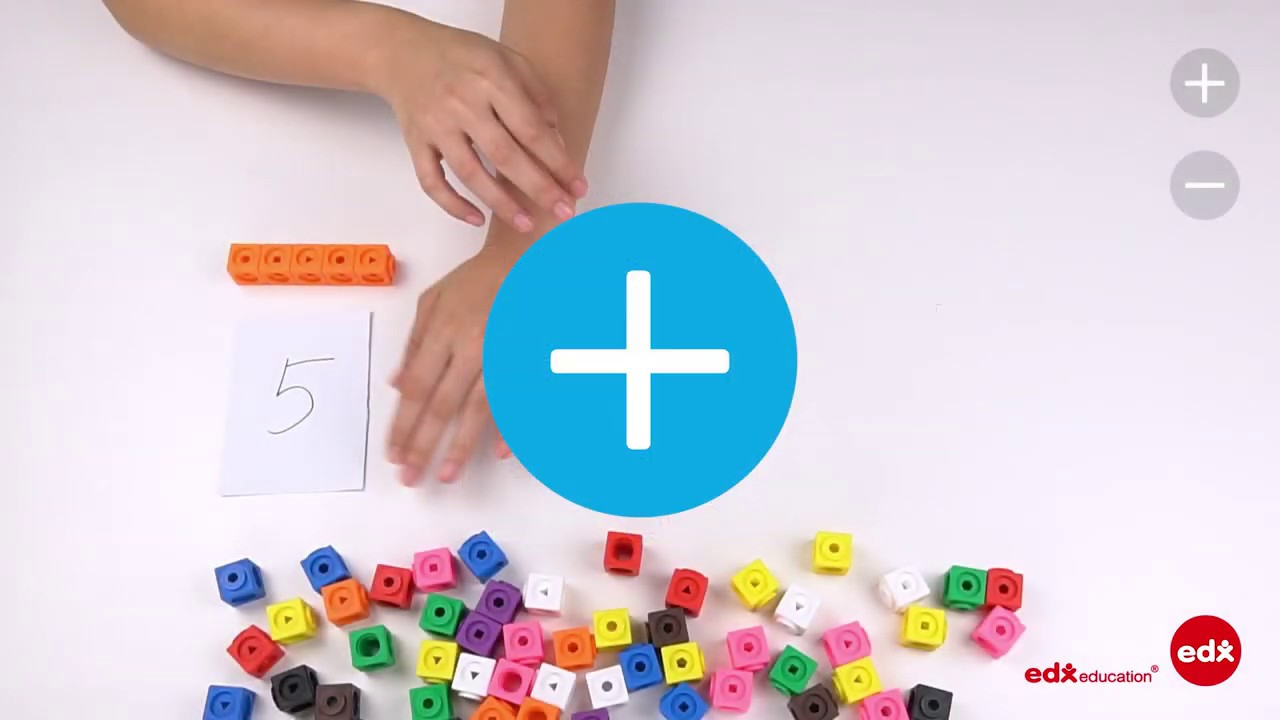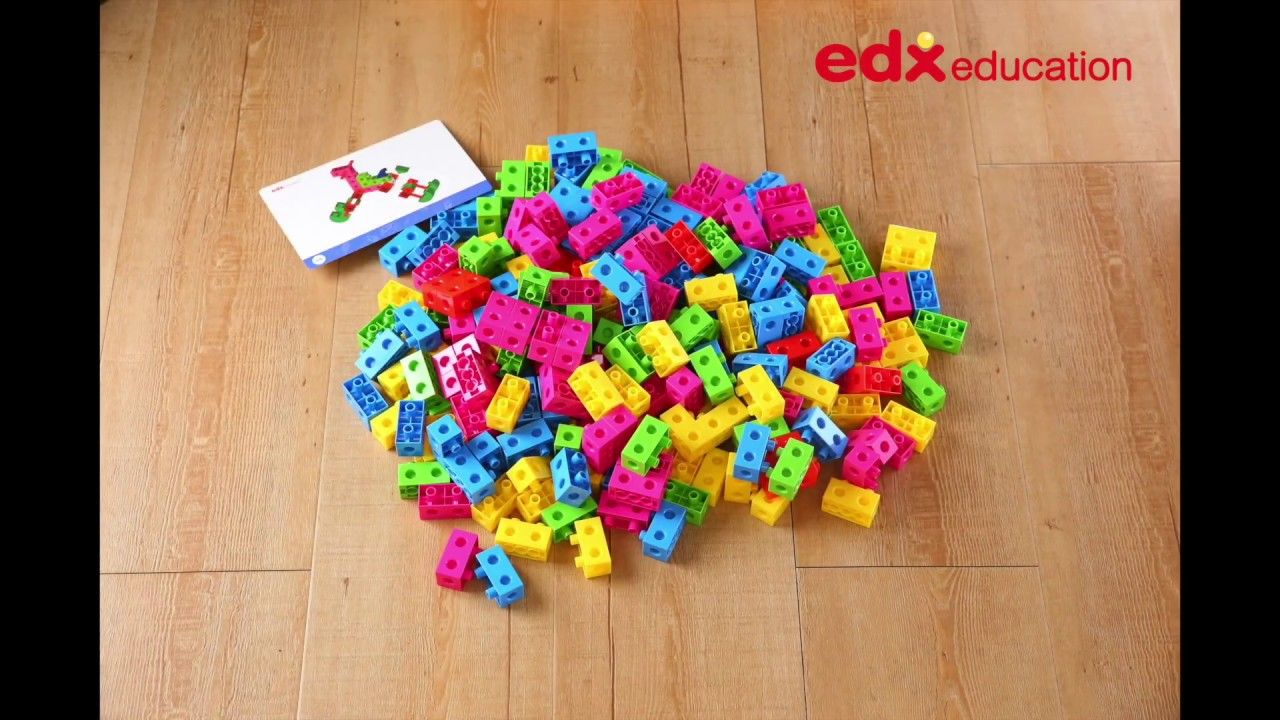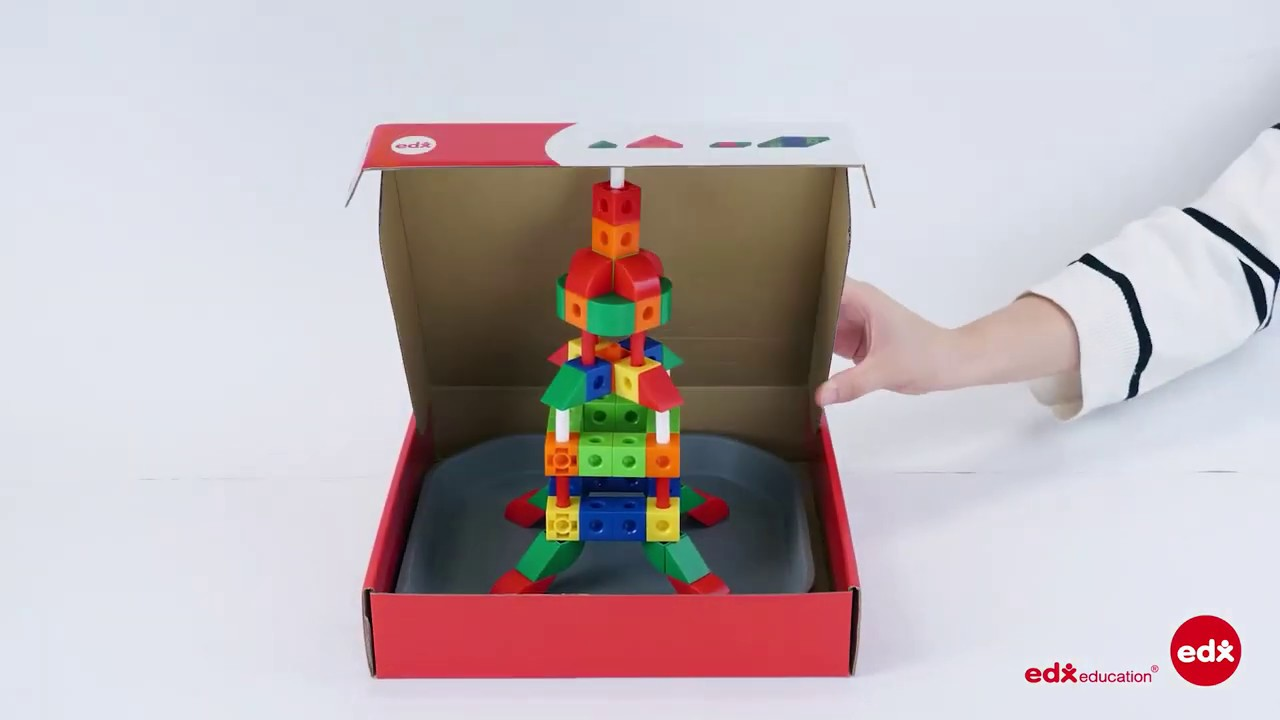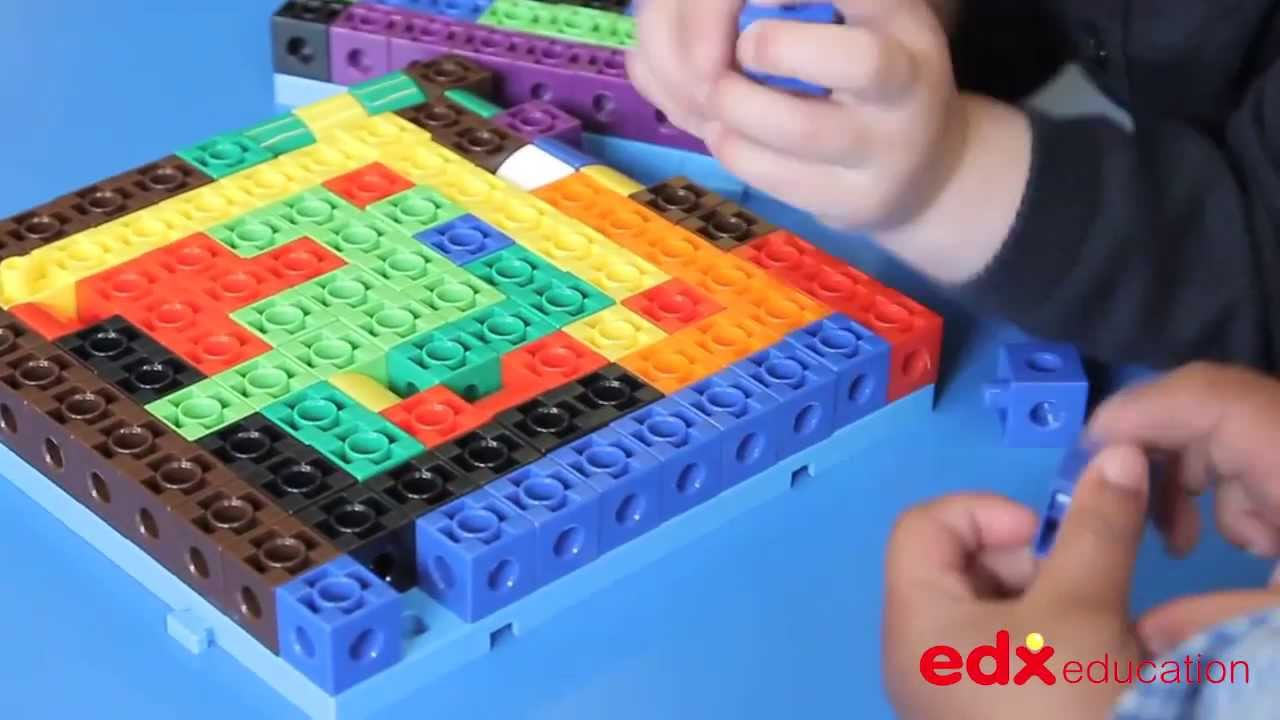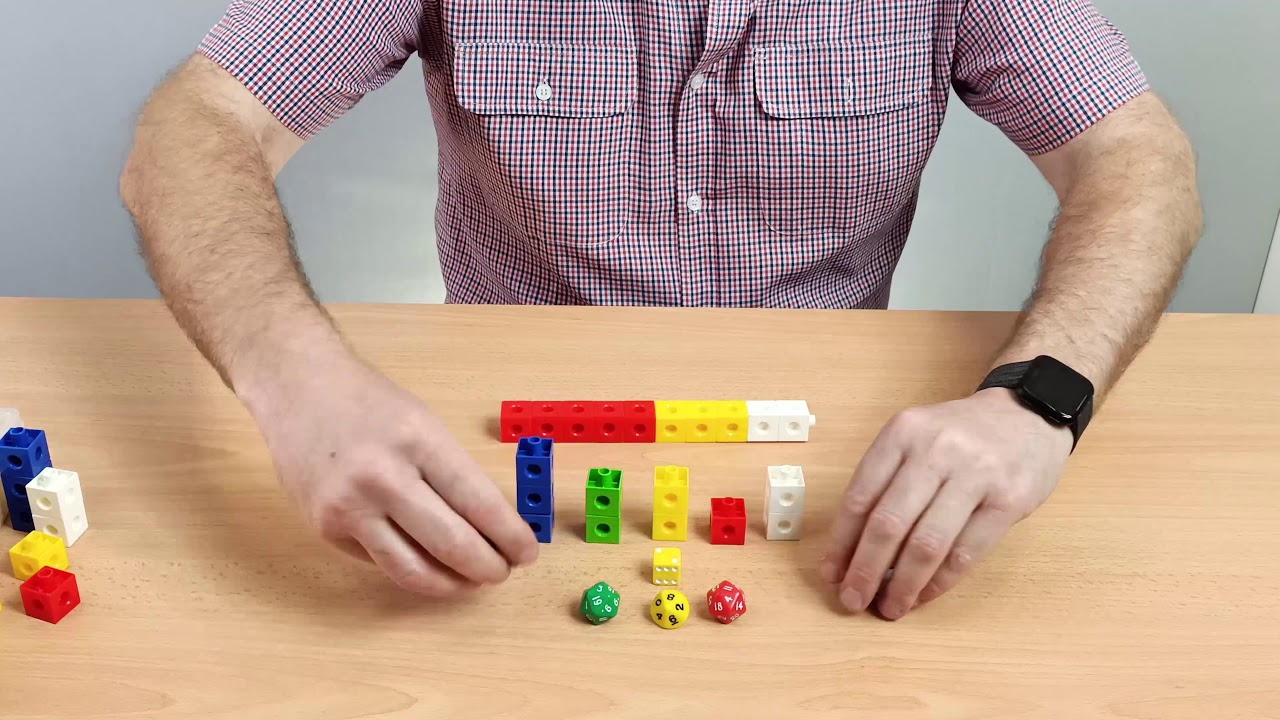### Mathematical Language

Edges, faces and vertices. Note that a cube may be referred to as a square based prism or a hexahedron.
Other language: longer, shorter, multiple.

The cubes may be linked to illustrate different mathematical language.
• Longer, shorter and the same/different length

### Using Cubes

#### Number

– Counting by multiples e.g. 3

##### Make A Number

– Make a Number – Use two different coloured cubes to show the ways of making a particular number. For example make five using two colours– (2 orange cubes and 5 red cubes joined together makes 7 cubes)##### Subtraction

– Snap a stick of 7 cubes into two pants,
e.g. 6 and 1             7-1=6,            7-6=1

#### Algebra - Patterning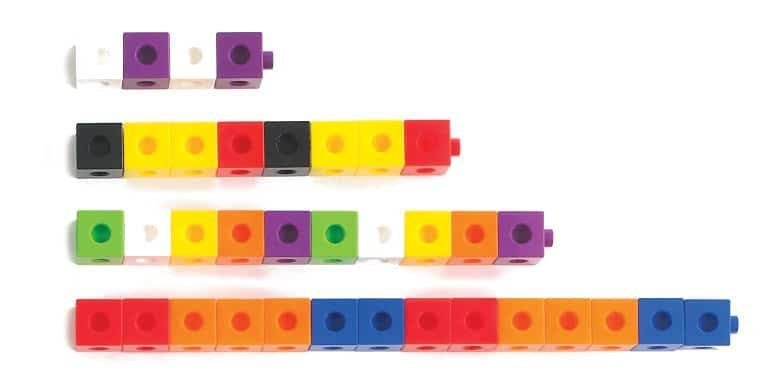#### Geometry

– The cubes link on all six faces which allows them to be made into various models. The ‘L’ shape may be rotated into different positions.

Consider all of the different models that may be made by joining four cubes.
Students may sketch the models on isometric dot paper, or by drawing the top view, front view and side view of the models that they make.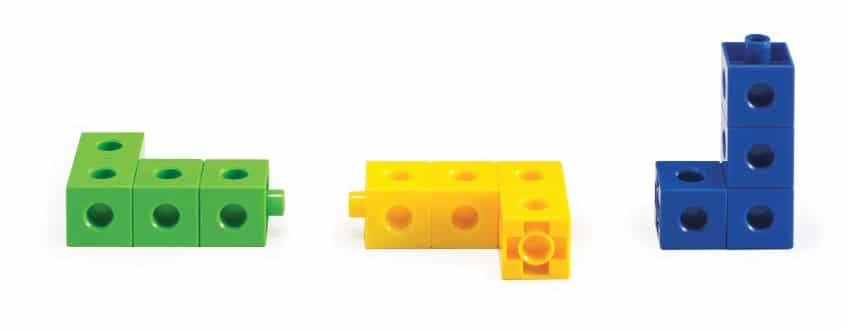#### Developing Spatial Reasoning

A typical task involving multilink type cubes would involve using four cubes to make all of the different possible models that can be made. This task involves a great deal of spatial reasoning as the builders will have to decide whether their models are unique or simply transformations
(flips, slides, turns) of each other.

#### Measurement - volume And Surface Area

Wooden or plastic 2 cm cubes may be used to fill boxes to determine the volume of a box. In this case the cube is deemed as the ‘unit’ and the volume would be recorded as a certain number of cubes. Remember the volume of a 2 cm cube is actually 2 cm x 2 cm x 2 cm or 8 cubic centimetres. If you want to measure the volume of a container in cubic centimetres then you will have to use cubiccentimeter blocks with smooth edges (eg the small cube that comes with a MAB set). The surface area would be found by counting the number of faces that were showing.

#### Probability

Placing an assortment of coloured cubes into a calico bag provides the opportunity of conducting probability experiments. Students can draw cubes out of the bag and then replace them to gather data.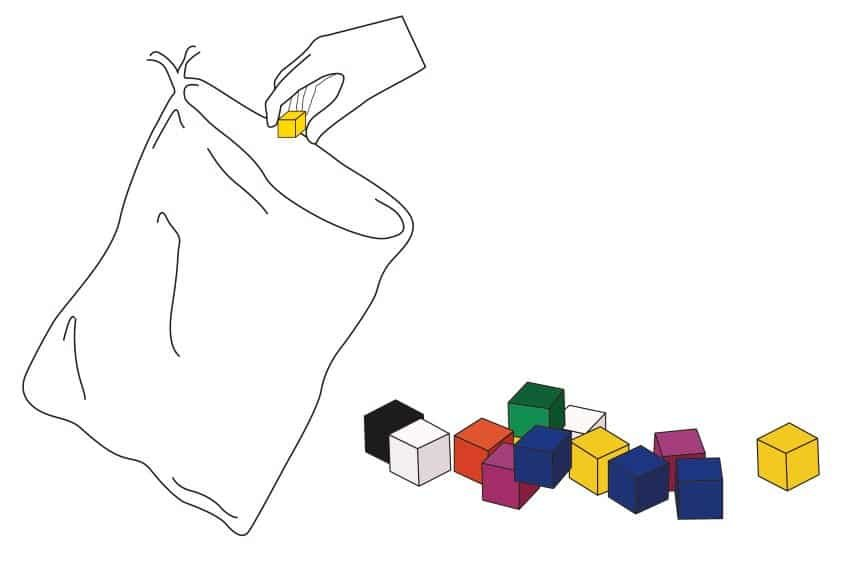### Typical Classroom Requirements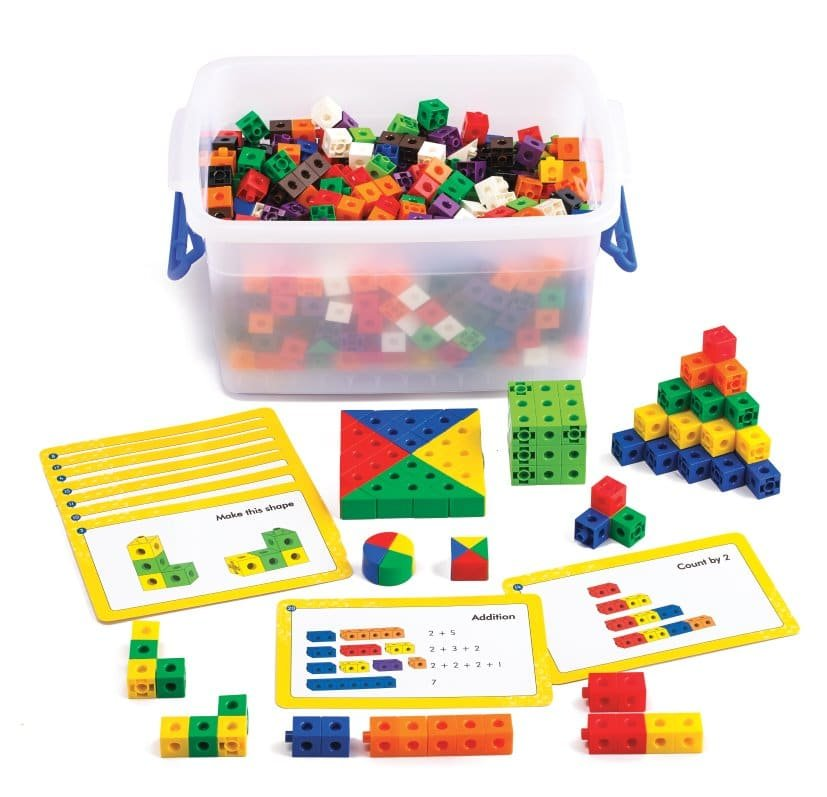#### class set:

• 3000 Linking Cubes (that is 100 per student)
• 2000 centicubes